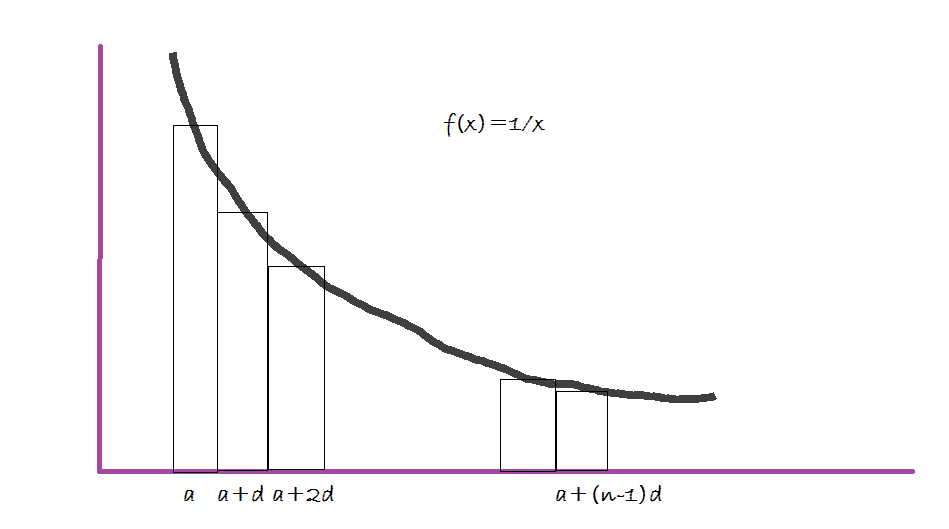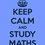# Sum of Harmonic Series

It is well known that the sum of a harmonic series does not have a closed form. Here is a formula which gives us a good approximation.

We need to find the sum of the following series

$\dfrac{1}{a}+\dfrac{1}{a+d}+\dfrac{1}{a+2d}+\ldots+\dfrac{1}{a+(n-1)d}$

Consider the function $f(x)=\frac{1}{x}$, we intend to take middle riemann sums with rectangles of width $d$ starting from $x=a$ to $x=a+(n-1)d$.Each rectangle in the figure has a width $d$. The height of the $i\text{th}$ rectangle is $\frac{1}{a+(i-1)d}$. The sum of the area of the rectangles is approximately equal to the area under the curve.

Area under f(x) from $x=a-\frac{d}{2}$ to $x=a+\left(n-\frac{1}{2}\right)d \approx\displaystyle\sum_{n=1}^{n} \frac{d}{a+(n-1)d}$

$\large\Rightarrow \int_{a-\frac{d}{2}}^{a+\left(n-\frac{1}{2}\right)d} \dfrac{\mathrm{d}x}{x}\approx \displaystyle\sum_{n=1}^{n} \frac{d}{a+(n-1)d}$

Let $S_n =\displaystyle\sum_{n=1}^{n} \frac{1}{a+(n-1)d}$

$\large\ln\dfrac{2a+(2n-1)d}{2a-d}\approx d\times S_n$

$\large\boxed{\Rightarrow s_n\approx\dfrac{\ln\dfrac{2a+(2n-1)d}{2a-d}}{d}}$

Note

• Apologies for the shabby graph.

• $d\neq 0$Note by Aneesh Kundu
5 years, 9 months ago

This discussion board is a place to discuss our Daily Challenges and the math and science related to those challenges. Explanations are more than just a solution — they should explain the steps and thinking strategies that you used to obtain the solution. Comments should further the discussion of math and science.

When posting on Brilliant:

• Use the emojis to react to an explanation, whether you're congratulating a job well done , or just really confused .
• Ask specific questions about the challenge or the steps in somebody's explanation. Well-posed questions can add a lot to the discussion, but posting "I don't understand!" doesn't help anyone.
• Try to contribute something new to the discussion, whether it is an extension, generalization or other idea related to the challenge.

MarkdownAppears as
*italics* or _italics_ italics
**bold** or __bold__ bold
- bulleted- list
• bulleted
• list
1. numbered2. list
1. numbered
2. list
Note: you must add a full line of space before and after lists for them to show up correctly
paragraph 1paragraph 2

paragraph 1

paragraph 2

[example link](https://brilliant.org)example link
> This is a quote
This is a quote
    # I indented these lines
# 4 spaces, and now they show
# up as a code block.

print "hello world"
# I indented these lines
# 4 spaces, and now they show
# up as a code block.

print "hello world"
MathAppears as
Remember to wrap math in $$ ... $$ or $ ... $ to ensure proper formatting.
2 \times 3 $2 \times 3$
2^{34} $2^{34}$
a_{i-1} $a_{i-1}$
\frac{2}{3} $\frac{2}{3}$
\sqrt{2} $\sqrt{2}$
\sum_{i=1}^3 $\sum_{i=1}^3$
\sin \theta $\sin \theta$
\boxed{123} $\boxed{123}$

Sort by:

Thanks! This is a very good and useful note.

- 5 years, 9 months ago

Thanks. :)

- 5 years, 9 months ago

Hey how you have assigned limit of $x$ can you please clarify

- 5 years, 9 months ago

$x$ varies from $a-\frac{d}{2}$ to $a+\left(n-\frac{1}{2}\right)d$.

- 5 years, 9 months ago

yaa ,I got this but also you can't use this formula for finding sum of similar terms

i.e. $S_n= \frac {1}{2}+ \frac {1}{2}+ \frac {1}{2}+ \frac {1}{2}+.... \frac {1}{2}(n^{th} term)$ as common difference is $0$ so it will be in indeterminate form

- 5 years, 9 months ago

Thanks for the suggestion, I added this point in the note.

- 5 years, 9 months ago

by the way is it real???

- 5 years, 9 months ago

This formula gives really good approximations when $d\rightarrow 0$ or for large values of $n$ with a not so big $d$ .

- 5 years, 9 months ago

Yes Absolutely

- 5 years, 9 months ago

Just followed you :-)

- 5 years, 9 months ago

I mean to is it original(your own)???

- 5 years, 9 months ago

Nope, its not purely original. I was reading about the convergence tests and I happened came across the Integral test, which inspired this note.

- 5 years, 9 months ago

it's really fantastic

- 5 years, 9 months ago

Thanks. :)

- 5 years, 9 months ago

your above expression will be incorrect when $\boxed{2a=d}$

- 5 years, 9 months ago

In this case calculate the sum from $a_2$ to $a_n$, using the given formula and then add $a_1$ to both sides.

- 5 years, 9 months ago

How can area under that curve=d×Sn ??here d is denoted as width

- 5 years, 5 months ago

Area under the curve $A=\frac{1}{a} d +\frac{1}{a+d} d+ \frac{1}{a+2d} d \ldots$ $\frac{A}{d}= ( \frac{1}{a}+ \frac{1}{a+d} \ldots )$ $A=d\dot S_{n}$

- 5 years, 5 months ago

Ohoo now i understand clearly. Thanks bro...

- 5 years, 5 months ago

@Aneesh Kundu I have just added your formula to Harmonic Progression wiki. I have also added important points from your discussion with Atul. You can also contribute to the wiki.

- 5 years, 4 months ago